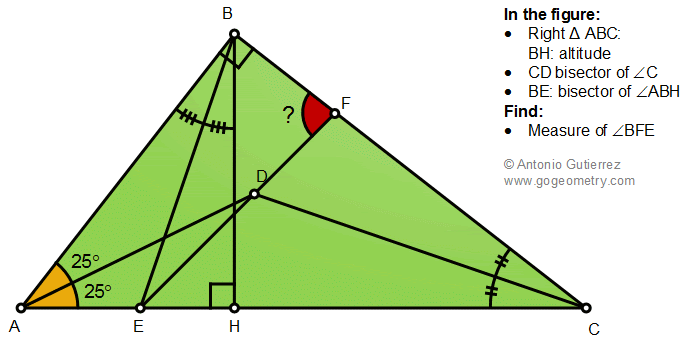## Wednesday, December 2, 2020

### Geometry Problem 1488: Right Triangle, Altitude, Angle Bisectors, Measurement

Geometry Problem 1488. Post your solution in the comment box below.
Level: Mathematics Education, K-12 School, Honors Geometry, College.

Details: Click on the figure below.1.FEC=45, C=40 => EFC=85 => EFB=95

2.https://photos.app.goo.gl/cA2eVoGjetr1yqXc7
from the result of problem 1487 we have CM⊥BE and ∠ (ADM)= 45 and triangle ECB is isosceles
in triangle BDC, external angle ∠BDM)= ∠ (DCB)+ ∠ (BDC)= 65
but ∠ (EDM)= ∠ (BDM)= 65 ( symmetric in isosceles triangle EBC)
3.